# signs

library(scales)
library(signs)
library(dplyr)
library(ggplot2)
library(ggrepel)

## The Basics

Using signs is simple, especially if you’re familiar with functions like number(), number_format(), comma(), comma_format(), percent(), and percent_format() from the scales package. It simply provides two new functions to complement these: signs() and signs_format().

• Points in group 1 are labeled with a true Unicode minus glyph.
• Points in group 2 are labeled with the traditional ASCII hyphen-minus.
• Basic usage is identical.
theme_set(theme_gray())
theme_update(
panel.grid.minor = element_blank(),
axis.text.y      = element_blank(),
axis.ticks.y     = element_blank()
)

p <-
ggplot(sleep) +
aes(group, extra) +
geom_point() +
xlab("Drug") +
ylab("Extra Sleep (hours)")

label_hours <- function(mapping) {
geom_text_repel(
mapping,
nudge_x       = -.1,
direction     = "y",
segment.size  = .4,
segment.color = "grey75",
hjust         = "right"
)
}

p +
label_hours(
aes(
label = case_when(
group == 1 ~ signs(extra, accuracy = .1),
group == 2 ~ number(extra, accuracy = .1)
)
)
)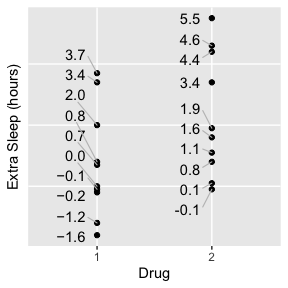## Other Number Formats

You can use any formatting function with signs::signs()—not just scales::number(). Let’s assume everyone gets 8 hours of sleep, so we can label the points as percentages.

p +
ylab("Extra Sleep (% over 8 hours)") +
label_hours(
aes(
label = case_when(
group == 1 ~ signs(extra / 8, accuracy = .1, format = scales::percent),
group == 2 ~ percent(extra / 8, accuracy = .1)
)
)
)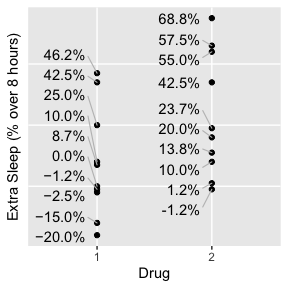Or we can muliply by days in a year and use scales::comma().

p +
ylab("Extra Sleep (hours / year)") +
label_hours(
aes(
label = case_when(
group == 1 ~ signs(extra * 365, format = scales::comma),
group == 2 ~ comma(extra * 365)
)
)
)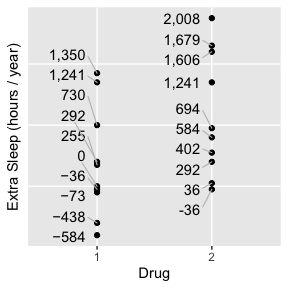format can be any function that takes a numeric vector and returns a character vector.

### Matching by Position

Note that format and all other options (see below) come after the dots; this way you can enjoy the same matching by position you know from scales. For example, if you prefer the simplicity of number(x, 1), you can simply use signs(x, 1).

x <- seq(-4, 4)

number(x, 1) # first argument is accuracy
#>  "-4" "-3" "-2" "-1" "0"  "1"  "2"  "3"  "4"
signs(x, 1)  # first argument is accuracy
#>  "−4" "−3" "−2" "−1" "0"  "1"  "2"  "3"  "4"

## Other Arguments

signs::signs() offers 3 other arguments for convenience:

1. add_plusses
2. trim_leading_zeros
3. label_at_zero

(#3 is addressed below under Axis Labels.)

Sometimes, as with this dataset, you want to show change from a baseline. You might not only want to include a − in front of negative numbers, but a + in front of positive numbers as well. This is as simple as add_plusses = TRUE.

p +
label_hours(
aes(
label = case_when(
group == 1 ~ signs(extra, accuracy = .1, add_plusses = TRUE),
group == 2 ~ number(extra, accuracy = .1)
)
)
)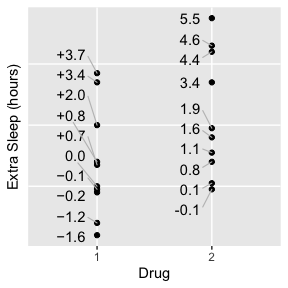If all values are on the interval (−1, 1), it can be more compact to remove leading zeros. Do this with trim_leading_zeros = TRUE:

p +
ylim(-.8, .8) +
label_hours(
aes(
label = case_when(
group == 1 ~ signs(extra, accuracy = .1, trim_leading_zeros = TRUE),
group == 2 ~ number(extra, accuracy = .1)
)
)
) +
theme(
axis.text.y = element_blank(),
axis.ticks.y = element_blank()
)
#> Warning: Removed 12 rows containing missing values (geom_point).
#> Warning: Removed 12 rows containing missing values (geom_text_repel).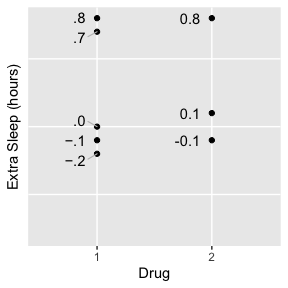## Axis Labels

You can also use Unicode minus signs on an entire axis. This function is called signs::signs_format(), by analogy to scales::number_format(), scales::percent_format(), and the rest of the _format() functions. Note that it accepts the same optional arguments as signs::signs() as well.

theme_update(
axis.text.y = element_text(hjust = 1)
)

p +
scale_y_continuous(
limits = c(-.8, .8),
breaks = seq(-.8, .8, by = .2),
labels = signs_format(
accuracy           = .1,
)
) +
label_hours(
aes(
label = case_when(
group == 1 ~ signs(
extra,
accuracy           = .1,
),
group == 2 ~ number(extra, accuracy = .1)
)
)
)
#> Warning: Removed 12 rows containing missing values (geom_point).
#> Warning: Removed 12 rows containing missing values (geom_text_repel).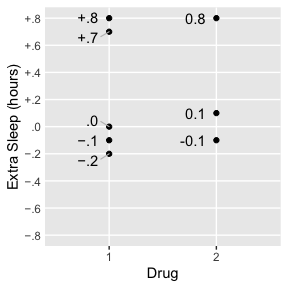You may want to treat zero itself differently, particularly when every other value has either a plus or a minus. Maybe you’ll be extra pedantic about it with label_at_zero = "symbol" (notice the y-axis labels below, not the data point labels):

p +
scale_y_continuous(
limits = c(-4, 6),
breaks = seq(-4, 6, by = 1),
labels = signs_format(
label_at_zero = "symbol"
)
) +
label_hours(
aes(
label = case_when(
group == 1 ~ signs(
extra,
accuracy = .1,
),
group == 2 ~ number(extra, accuracy = .1)
)
)
)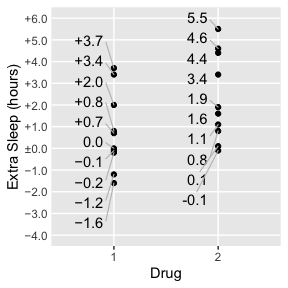Or, especially if the location of zero is already obvious, you might want to leave it blank with label_at_zero = "blank":

p +
scale_y_continuous(
limits = c(-4, 6),
breaks = seq(-4, 6, by = 1),
labels = signs_format(
label_at_zero = "blank"
)
) +
label_hours(
aes(
label = case_when(
group == 1 ~ signs(
extra,
accuracy = .1,
),
group == 2 ~ number(extra, accuracy = .1)
)
)
)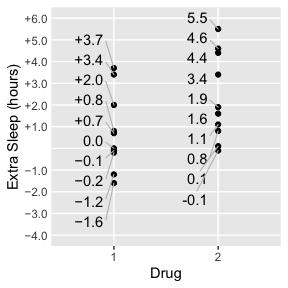## Setting Options Globally

You can set format, add_plusses, trim_leading_zeros, or label_at_zero globally for a script with options():

options(
signs.format             = scales::number,
signs.label.at.zero      = "none"
)

p +
scale_y_continuous(
limits = c(-.8, .8),
breaks = seq(-.8, .8, by = .2),
labels = signs_format(accuracy = .1, label_at_zero = "blank")
) +
label_hours(
aes(
label = case_when(
group == 1 ~ signs(extra, accuracy = .1),
group == 2 ~ number(extra, accuracy = .1)
)
)
)
#> Warning: Removed 12 rows containing missing values (geom_point).
#> Warning: Removed 12 rows containing missing values (geom_text_repel).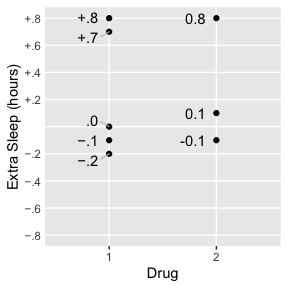The defaults are scales::number, FALSE, FALSE, and "none", respectively.